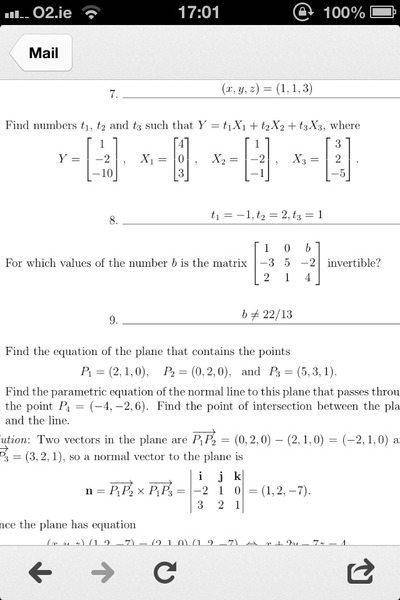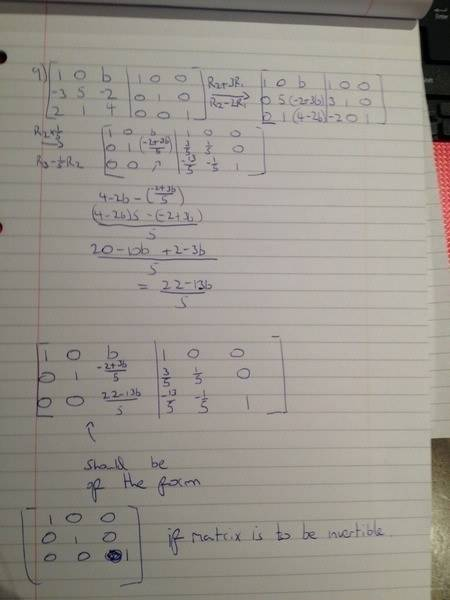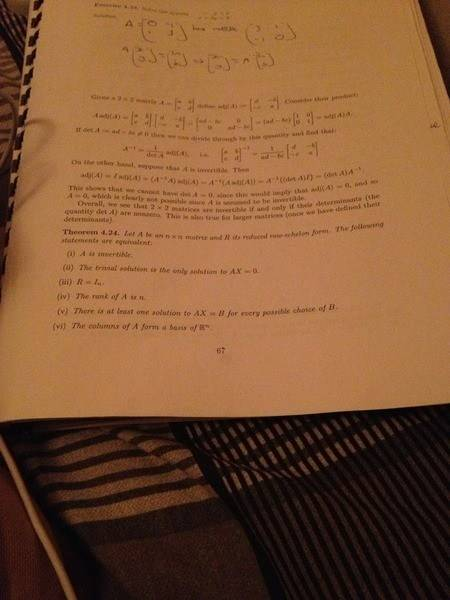# 3 x 3 matrix with unknowns. Conditions for invertibility.I've attached 2 images above. The first is the problem itself and the answer. It's question 9. So, I tried solving this question using the method of finding the inverse of a n x n matrix by augmenting it with the identity matrix of the same dimensions and then applying row ops in an attempt to change the matrix on the left of the augmentation (the given matrix) to the identity matrix. I then though I would have some conditions for b. I'm not sure if I've just made a mistake in my calculations or whether my general approach is off. Any help would be much appreciated.

## Answers and Replies

Ray Vickson
Science Advisor
Homework Helper
Dearly Missed
View attachment 56766View attachment 56767
I've attached 2 images above. The first is the problem itself and the answer. It's question 9. So, I tried solving this question using the method of finding the inverse of a n x n matrix by augmenting it with the identity matrix of the same dimensions and then applying row ops in an attempt to change the matrix on the left of the augmentation (the given matrix) to the identity matrix. I then though I would have some conditions for b. I'm not sure if I've just made a mistake in my calculations or whether my general approach is off. Any help would be much appreciated.

So far what you have looks OK, but you still have not answered the question (at least as far as I can make out). What values of b allow for inversion? What values give a matrix that is not invertible?

So far what you have looks OK, but you still have not answered the question (at least as far as I can make out). What values of b allow for inversion? What values give a matrix that is not invertible?

Well, b would have to be 0 for the top row, 2/3 for the middle row and 17/13 for the last row, for the matrix to be a 3 x 3 identity matrix and clearly it can't be all three at the same time. Does the above make sense or am I thinking about this in the wrong way??

DrClaude
Mentor
What is the condition on the determinant for a matrix to be invertible?

When it's determinant is non zero. There has to be a way to figure it out with row ops though. At the time of the exam we we hadn't come to matrix determinant. Is there any tips you can give me for doing it with row ops?

Just thinking there, for the matrix to be invertible it's rank must equal three, so the bottom row must be nonzero. So, b must NOT be equal to 22/13 for the bottom row to be nonzero. Yeah?

If this is true though, the matrix wouldn't be a n x n identity matrix due to the above entries in the third column being nonzero.

Ray Vickson
Science Advisor
Homework Helper
Dearly Missed
Just thinking there, for the matrix to be invertible it's rank must equal three, so the bottom row must be nonzero. So, b must NOT be equal to 22/13 for the bottom row to be nonzero. Yeah?

Yes.

And, to answer your next question: if b is not 22/13, there is a nonzero number in the (3,3) position. You can then add multiples of it back to rows 1 and 2 to get a unit matrix. If you don't do that---but just leave the non-zeros above the diagonal---you are doing so-called Gaussian elimination. If you also zero-out the elements above the diagonal, you are doing Gauss-Jordan elimination.

Basically, you do Gaussian elimination when you want to solve equations and Gauss-Jordan elimination when you want to compute matrix inverses.

That's brilliant. Thanks! One final question and I'm done.In the attached image at the bottom of the page there are the conditions, of sorts, for invertibility. The statement "The following statements are equivalent" does this mean that if one of these statements are true that the rest are also true? Thanks for all your help.